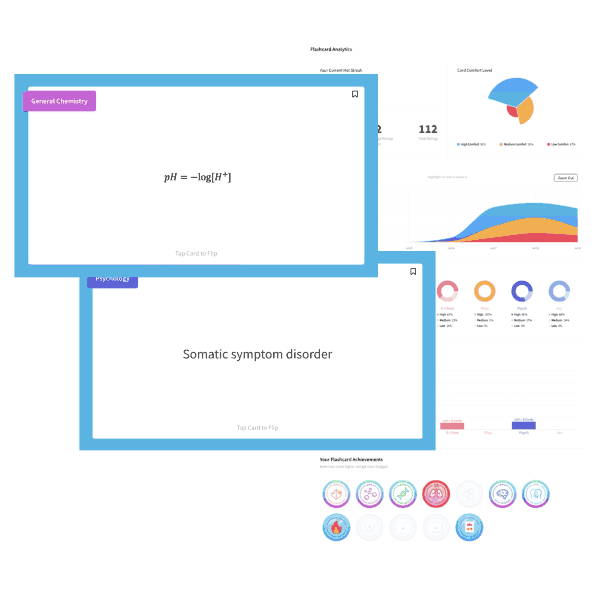# MCAT Biology Question — Genotypes

What proportion of offspring with the genotype AABBCC will result from a trihybrid cross for the unlinked genes A, B, and C?

1. 1/8
2. 1/16
3. 1/32
4. 1/64
##### Click for Explanation

This question tests your ability to calculate the probability of a possible genotype resulting from a trihybrid cross between two heterozygotes. One method to determine the probability is to make a Punnett square. An organism that is heterozygous for three genes can produce eight different gamete possibilities. Thus, resultant Punnett square would contain an 8×8 table with a total of 64 genotype combinations. A more efficient method is to calculate the probability of each event occurring separately and then use the rule of multiplication to determine the likelihood of all three events occurring at the same time.

Probability of obtaining an AA genotype from a cross between Aa and Aa = ¼

Probability of obtaining a BB genotype from a cross between Bb and Bb = ¼

Probability of obtaining a CC genotype from a cross between Cc and Cc = ¼

Total probability of all three possibilities = ¼ x ¼ x ¼ = 1/64

Therefore, D is the correct answer.

## Want more MCAT practice?

### We’ve got options for every schedule and learning style!

From the best online MCAT course created by top instructors with 524+ MCAT scores to the most representative full-length practice exams and private tutoring, we can custom tailor your MCAT prep to your goals!

Not sure which option is right for you? Schedule a free MCAT consultation with an MCAT expert using the form below. No obligation, just expert advice.

Create your Free Account to access our MCAT Flashcards